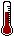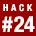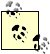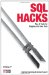# Hack 24. Multiply Across a Result SetWith certain calculations, such as compound interest, you need to multiply a set of values. How come there's no PRODUCT aggregate function that is to multiplication as SUM is to addition?

SQL has no aggregate function for multiplication, but you can use logarithms to achieve the desired result. When you add the logarithms of a list of numbers you get the same result you would get if you had taken the logarithm of their product:

log(a) + log(b) + log(c) = log(a*b*c)

The inverse of the logarithm is the exponent function:

exp(log(a) + log(b) + log(c)) = a*b*c

So, to multiply the values 3, 4, and 5 without using multiplication, you could do the following:

```mysql> select exp( ln(3)+ln(4)+ln(5) );
+------------------------+
| exp(ln(3)+ln(4)+ln(5)) |
+------------------------+
| 60 |
+------------------------+```

You can also use this technique to achieve the same effect as a PRODUCT( ) aggregate function. Suppose you have invested \$100 in a savings account that has produced the interest rates shown in Table 5-1.

Table 5-1. Interest rates by year

yr rate
2002 5%
2003 4%
2004 5%
2005 3%

The effective rate over the four years is not 5% + 4% + 5% + 3% = 17%. Although that's an approximation in the short term, it is not accurate because it does not compound the interest. Instead, you need to multiply the factors. So the calculation must be 1.05x1.04x1.05x1.03 = 1.180998 (18.0998%).

You can calculate the multiplier and its logarithm easily in SQL:

```mysql> SELECT yr,
-> 1+rate/100 AS factor,
-> ln(1+rate/100) AS log
-> FROM interest;
+------+--------+-------------------+
| yr | factor | log |
+------+--------+-------------------+
| 2002 | 1.05 | 0.048790164169432 |
| 2003 | 1.04 | 0.039220713153281 |
| 2004 | 1.05 | 0.048790164169432 |
| 2005 | 1.03 | 0.029558802241544 |
+------+--------+-------------------+```

To determine the effective rate you need to sum the log columnthat will give the logarithm of the cumulative factor column:

```mysql> SELECT SUM(LN(1+rate/100)) FROM interest;
+---------------------+
| SUM(LN(1+rate/100)) |
+---------------------+
| 0.16635984373369 |
+---------------------+```

This is still just the logarithm, so now you need to calculate its inverse, or take the exponent to get the product:

```mysql> SELECT EXP(SUM(LN(1+rate/100))) FROM interest;
+--------------------------+
| EXP(SUM(LN(1+rate/100))) |
+--------------------------+
| 1.180998 |
+--------------------------+```

You can multiply this by your investment (\$100) to find out how much you're worth now:

```mysql> SELECT EXP(SUM(LN(1+rate/100)))*100 FROM interest;
+------------------------------+
| EXP(SUM(LN(1+rate/100)))*100 |
+------------------------------+
| 118.0998 |
+------------------------------+```

You've made 18 bucks plus change!The natural logarithm function is called LN in Oracle, PostgreSQL, and MySQL. In SQL Server and Access, the logarithm function is called LOG rather than LN. Otherwise, everything is the same across database platforms.SQL Hacks
ISBN: 0596527993
EAN: 2147483647
Year: 2004
Pages: 147

Similar book on Amazon